Email : info@epmstrategy.com | Call Us Now : 479-321-3977
:: Home :: Sitemap ::

# Power BI – Use Join DAX Functions

BY: David Rohlfs

Cross Join

Natural Inner Join

Natural Left Outer Join

Introduction

There are a lot of reasons that you need to incorporate multiple tables into one inside of Microsoft Power BI Desktop. And a few of the ways that you can achieve this are by using DAX. There are a few functions that we will be going over in this blog to represent the Join Functions. These functions will be CrossJoin(), NaturalInnerJoin(), and NaturalLeftOuterJoin().

In this blog, we will go over when to use the Join functions, some common problems that you may see when using these functions, and a walkthrough that shows you how to use these functions.

Before you read through this blog, I suggest that you go and read through the Append and Merge blogs by EPM Strategy. Doing this will give you a good understanding of what is happening with the comparisons in this blog. The link to some of the blogs and articles you may be interested in will be at the end of the page.

When to use it

The Cross Join, Natural Inner Join, and Natural Left Outer Join DAX functions are a way that you can avoid using Power Query to merge or append your tables and data. While the three functions are similar in that they are “Joining” your tables together, there are some subtleties and nuances to each of them.

One thing that you should keep in mind is that the join functions are only aimed to take the tables and join them together. This means that you are taking the entire table and trying to attach it to another table. If there are columns that have errors in them, or column names that do not follow the respective requirements (i.e., two columns must have the same or different names), then the new table function may not work.

There are some strong reasons why you may like or dislike the idea of using a Join function. Some pros would be:

• If you already know DAX,P then learning another function is not much work.
• A DAX function may be less performance reducing than using m-code.
• You may have restrictions on power query and prefer to use DAX to merge two tables together.

Some cons may be:

• The next person that edits this report may not know DAX well enough to understand what is happening with the function.
• Depending on your dataset, a DAX function may not be as considering of performance as using m-code.
• DAX is much harder to learn for most people and may not be the best choice for many reports.

For the Cross Join Function:

The goal of this function is to join two or more tables together in a merge. To do this, the DAX function follows a Cartesian Product that joins every row of one table with every row of another table.

Here is a visual to illustrate this: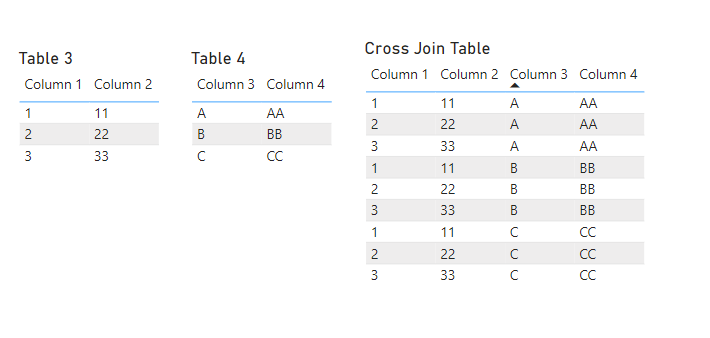In this function you are taking every row of one table and corresponding it with every row of another table. For many situations, this won’t be helpful because it is repeating data points in each of the columns present. But this could also be exactly what you want to show on a table for your end user.

For Example: If you chose to use this function on Table A that has five rows and Table B that has three rows, then you would pair each of the rows from Table A to all the rows from Table B. This would make a total of 15 rows because of the five rows from Table A multiplied by the three rows from Table B.

For the Natural Inner Join Function:

This Function will work more similarly than the CrossJoin to a normal merge. There are some nuances that you will need to learn before using this function though. To start, let’s look at how this function works in the first place. It is very similar at first glance to a normal merge command in that it shows all the columns that you are joining together.

Here is a visual to visualize what the output for this function is: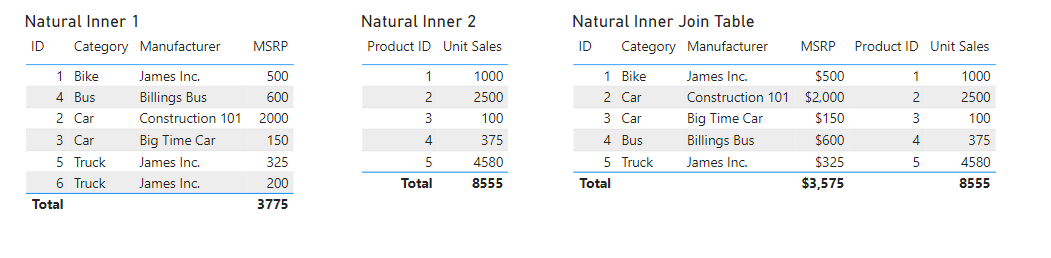The tricky part about this function is that you still need a column that is based on some sort of index or row specific value. At the end of this blog there is a link to go and read another EPM Strategy blog on this topic.

Normally having a relationship between these two tables wouldn’t be a problem at all, but the two fields cannot have the same name. In the screenshot above you can see that I have both a “Product ID” and “ID”. These are the same value and have a relationship connecting the two (As seen in the image below), but they do not have the same name. This is because you will get an error if both fields have the same name between the two tables (this isn’t just for the column with the relationship).

Here is what my relationship between the two tables looks like: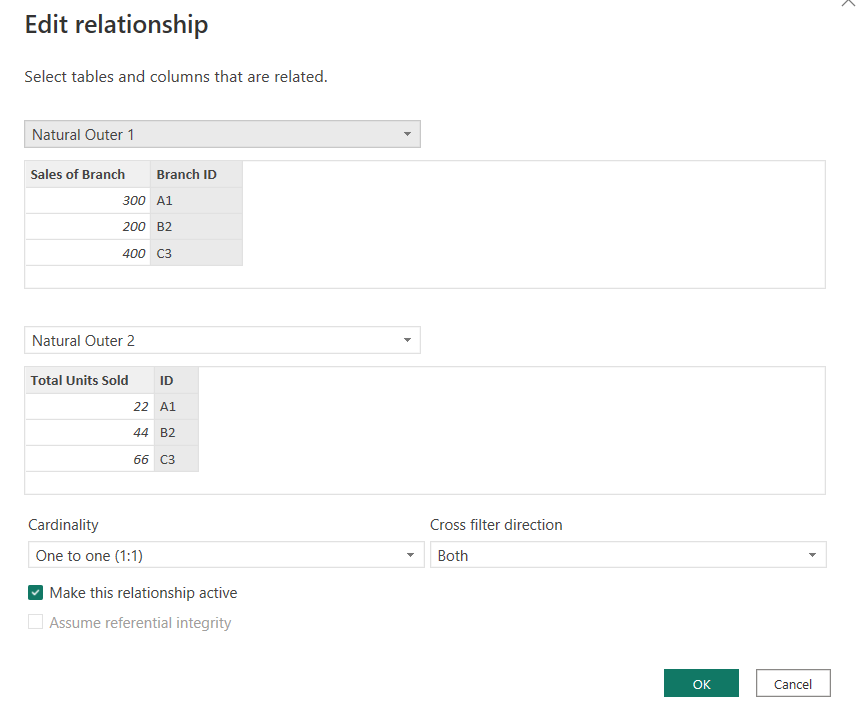For most cases you my need to mess around with the cardinality, but for this scenario a one to one worked just fine for me.

If you can get past the need for a row specific column that has different names, then this function does act very much like a normal merge.

For the Natural Left Outer Join Function:

Out of the three join functions, this Natural Left Outer Join function gave me the most trouble in completing and understanding. There are a lot more nuances with this function than first meet the eye, and because of that I want to go ahead and show you an example.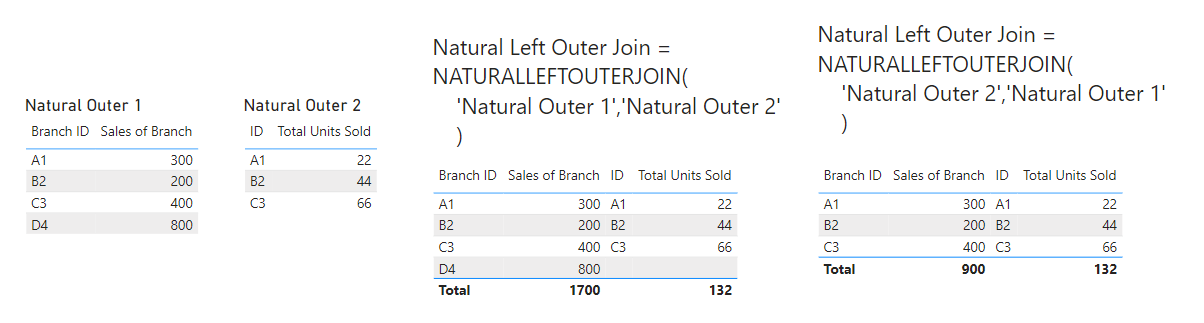In this image you see the two starting tables on the left. And on the right, you see the two tables that have the join function calculating them. Because of the order that we put the tables in, not all our rows showed up on the second table. This is because you are only taking the rows from the second table and attaching them to the rows from the first table. So, if I have 30 rows in Table A, and 35 rows in Table B, but only 20 of the rows from Table A match the rows from Table B, then I will get all the 30 rows from Table A and only the 20 rows from Table B (With the other rows being blank) in the new table.

When you are joining tables using this function, what you are doing is using all the first table entered, and only taking rows from the second table that have a relationship to the first. This is where some difficulties can come into play if you are using this function. You need to have a relationship between the two tables that have the same column values without having the same column name.

Note: On the Microsoft DAX website, this is not what is stated. In the scenario that I used for this blog I was receiving error messages and was not able to complete my equation when both columns had the same name. If you do not have the same name in both columns and you get an error message, you might want to try using the same name.

Common Problems

If a “Sub-Table” has an error, the New DAX Table will not work and will show an error.

For natural Inner Join:

You need to make sure that the column you are making your relationship with has a different name between the two tables. When first using this function, I spent a lot of time trying to find where my error was coming from and consistently overlooked this issue.

For Natural Left Outer Join:

If you read through the Microsoft DAX Guide (Link Below), then you’ll notice that it says you need to have common column names. As far as I can tell, this is entirely Incorrect. It took me a while to figure out but in the example that I came up with you need to have different names just like Natural Inner Join.

Walkthrough

Because this blog is covering DAX functions and not techniques or demonstrations, this walkthrough is going to mostly show what the functions will look like.

To start, I want to first show you how to create a DAX Table. First, you click on modeling, then on New Table.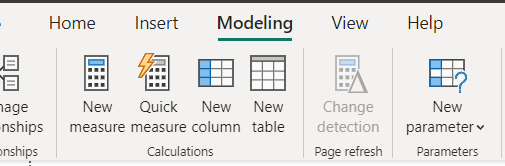For the formula you may choose to use variable statements, which could help when trying to create more complex tables, or when you want to select columns from tables before joining them together.

For all three formulas, I have thrown in some images below that show some of what they are doing. All the original tables that I used in the formula are on the left, and the tables that I created are on the right. For all the formulas you just type in the function(‘Table 1’, ‘Table 2’).

CrossJoin():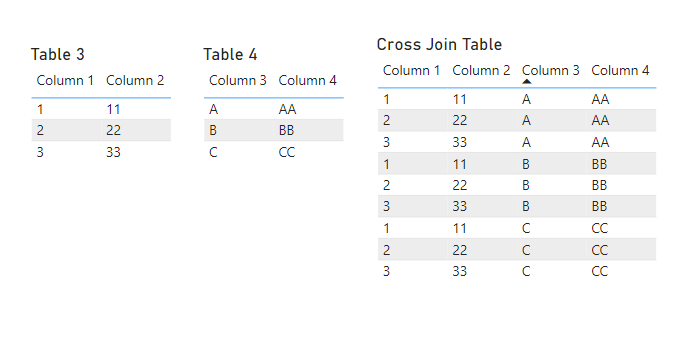NaturalInnerJoin():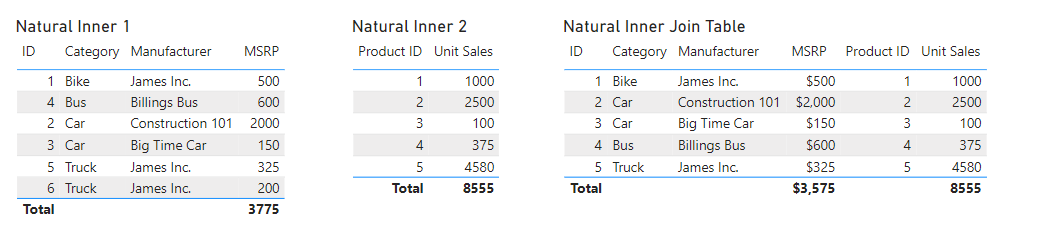NaturalLeftOuterJoin():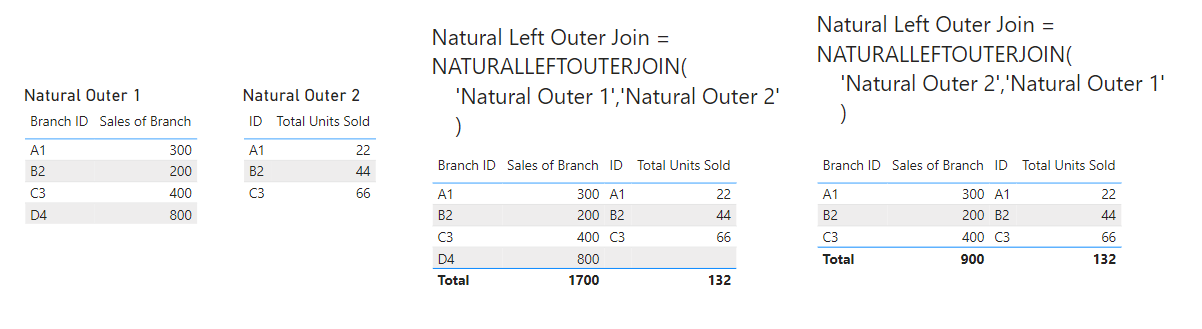Conclusion

All the Join functions can be very useful when trying to use DAX to create tables. There are a lot of difficulties when trying to learn them, but once you figure out some of the nuances, these functions can be a go to for many Power BI report builders.

Link to “How to Combine tables in Power BI”

https://learn.microsoft.com/en-us/dax/crossjoin-function-dax

https://learn.microsoft.com/en-us/dax/naturalinnerjoin-function-dax

https://learn.microsoft.com/en-us/dax/naturalleftouterjoin-function-dax

Power BI Row Specific Columns : :: Welcome To EPM Strategy ::

Power BI Understanding the Basics of Using Variables in DAX : :: Welcome To EPM Strategy ::